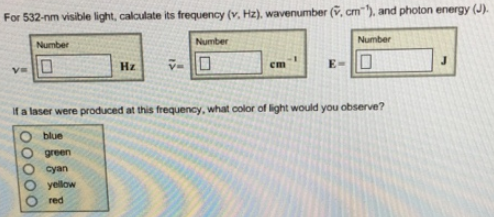# Problem: For 532-nm visible light, calculate its frequency (v, Hz), wavenumber (v, cm-1), and photon energy (J). If a laser were produced at this frequency, what color of light would you observe?

###### FREE Expert Solution
85% (485 ratings)###### Problem Details

For 532-nm visible light, calculate its frequency (v, Hz), wavenumber (v, cm-1), and photon energy (J).

If a laser were produced at this frequency, what color of light would you observe?